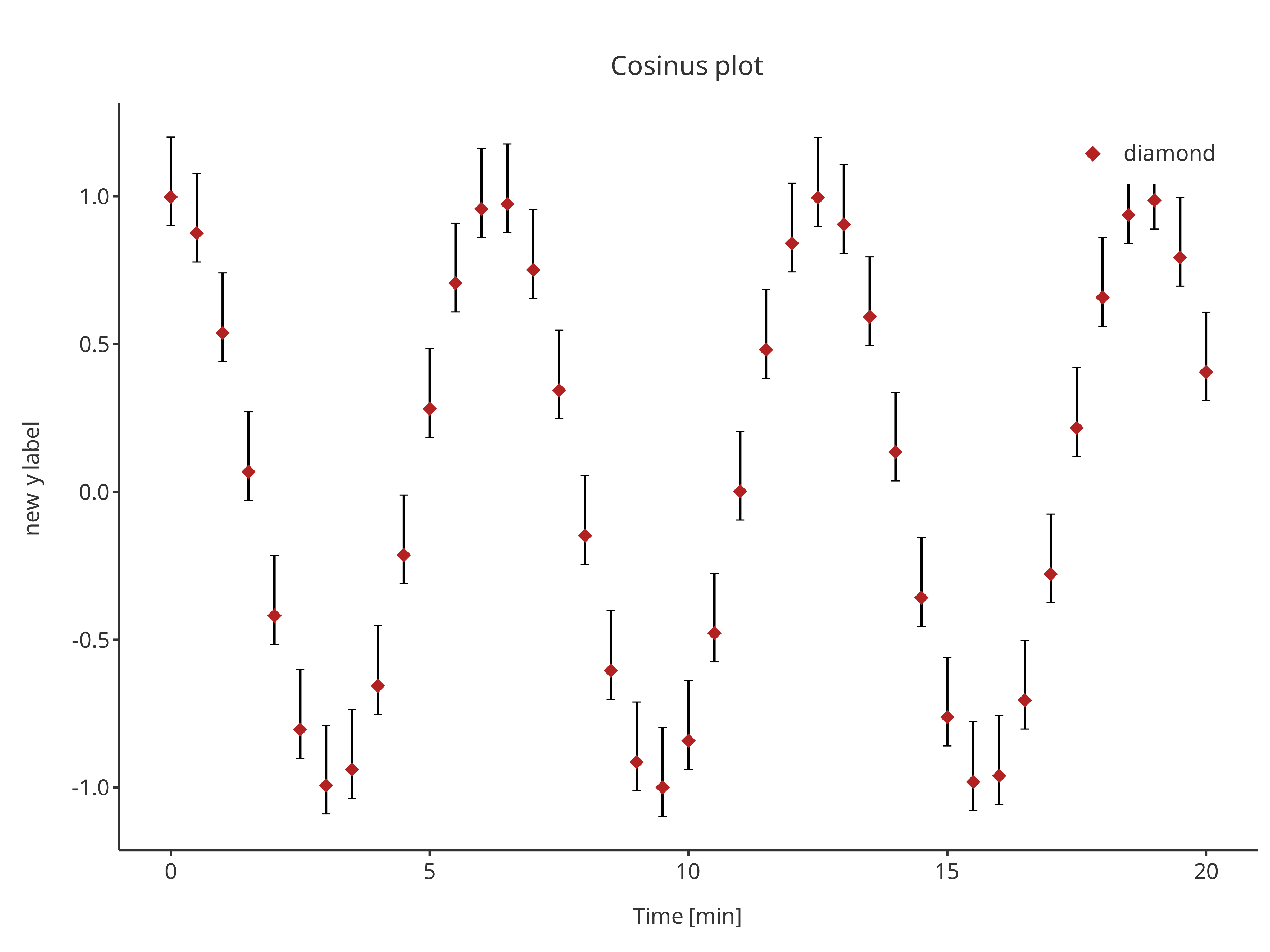require(tlf)
#> Loading required package: tlf

This vignette tackles how to use atom plots to prepare simple to more complex plots with the tlf-library.

## Introduction to atom plots

An atom plot corresponds to a simple plot that can be combined with other atom plots to form a final molecule plot, often more complex.

## initializePlot

The tlf function initializePlot creates an empty plot whose properties are defined by a PlotConfiguration object. The function initializePlot is called by every tlf plot function if no plotObject is provided as input and returns a regular ggplot object that includes an additional field named plotConfiguration defining its properties as a PlotConfiguration object.

A detailed presentation of PlotConfiguration objects is available in the vignette plot-configuration.

A few examples that use initializePlot are shown below.

• Initialize an empty plot from the current theme (no plotConfiguration input)
initializePlot()• Initialize an empty plot with labels defined by plotConfiguration input
# Create a PlotConfiguration object to specify plot properties (here labels)
myConfiguration <- PlotConfiguration$new( title = "My empty plot", xlabel = "My X axis", ylabel = "My Y axis" ) # Use the obect to define the plot properties initializePlot(plotConfiguration = myConfiguration)• Initialize an empty plot whose plotConfiguration input uses data, metaData and dataMapping to fill the plot x and y labels. • if metaData provides dimension and unit, the labels will be dimension [unit] by default. • if only data is provided, the labels will include the column name by default • if xlabel and ylabel are used, they will replace those default # data.frame for example knitr::kable(head(cosData)) time cos sin 0.0 1.0000000 0.0000000 0.5 0.8775826 0.4794255 1.0 0.5403023 0.8414710 1.5 0.0707372 0.9974950 2.0 -0.4161468 0.9092974 2.5 -0.8011436 0.5984721 # Define which is x and y variables using dataMapping cosMapping <- XYGDataMapping$new(x = "time", y = "cos")

# Define the metaData
time = list(
dimension = "Time",
unit = "min"
),
cos = list(
dimension = "Cosinus",
unit = ""
)
)
#> $time #>$time$dimension #>  "Time" #> #>$time$unit #>  "min" #> #> #>$cos
#> $cos$dimension
#>  "Cosinus"
#>
#> $cos$unit
#>  ""
# Define default Configuration with metaData
metaDataConfiguration <- PlotConfiguration$new( title = "Cosinus plot", data = cosData, metaData = cosMetaData, dataMapping = cosMapping ) initializePlot(plotConfiguration = metaDataConfiguration)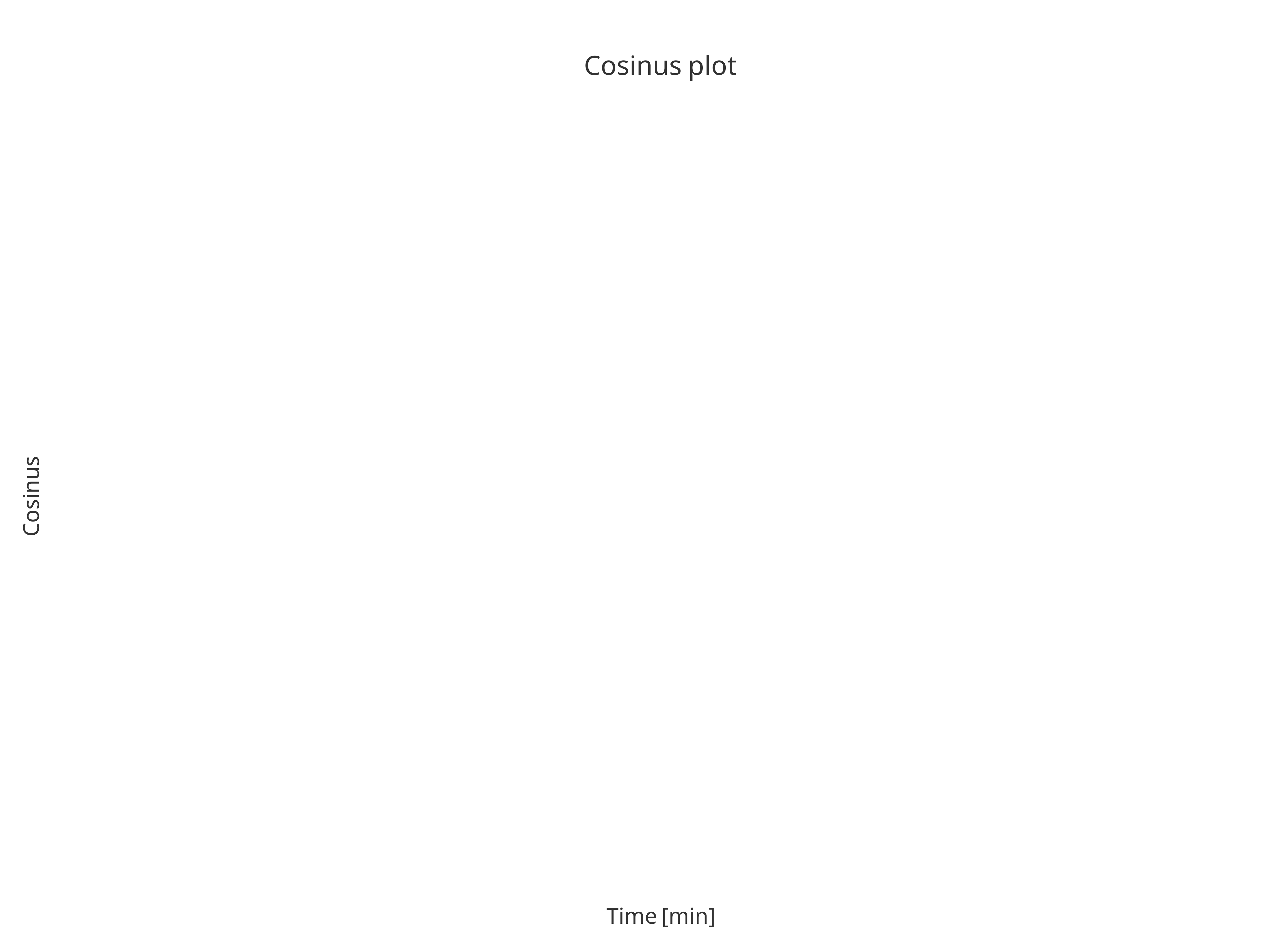# Define default Configuration with metaData dataConfiguration <- PlotConfiguration$new(
title = "Cosinus plot",
data = cosData,
dataMapping = cosMapping
)

initializePlot(plotConfiguration = dataConfiguration)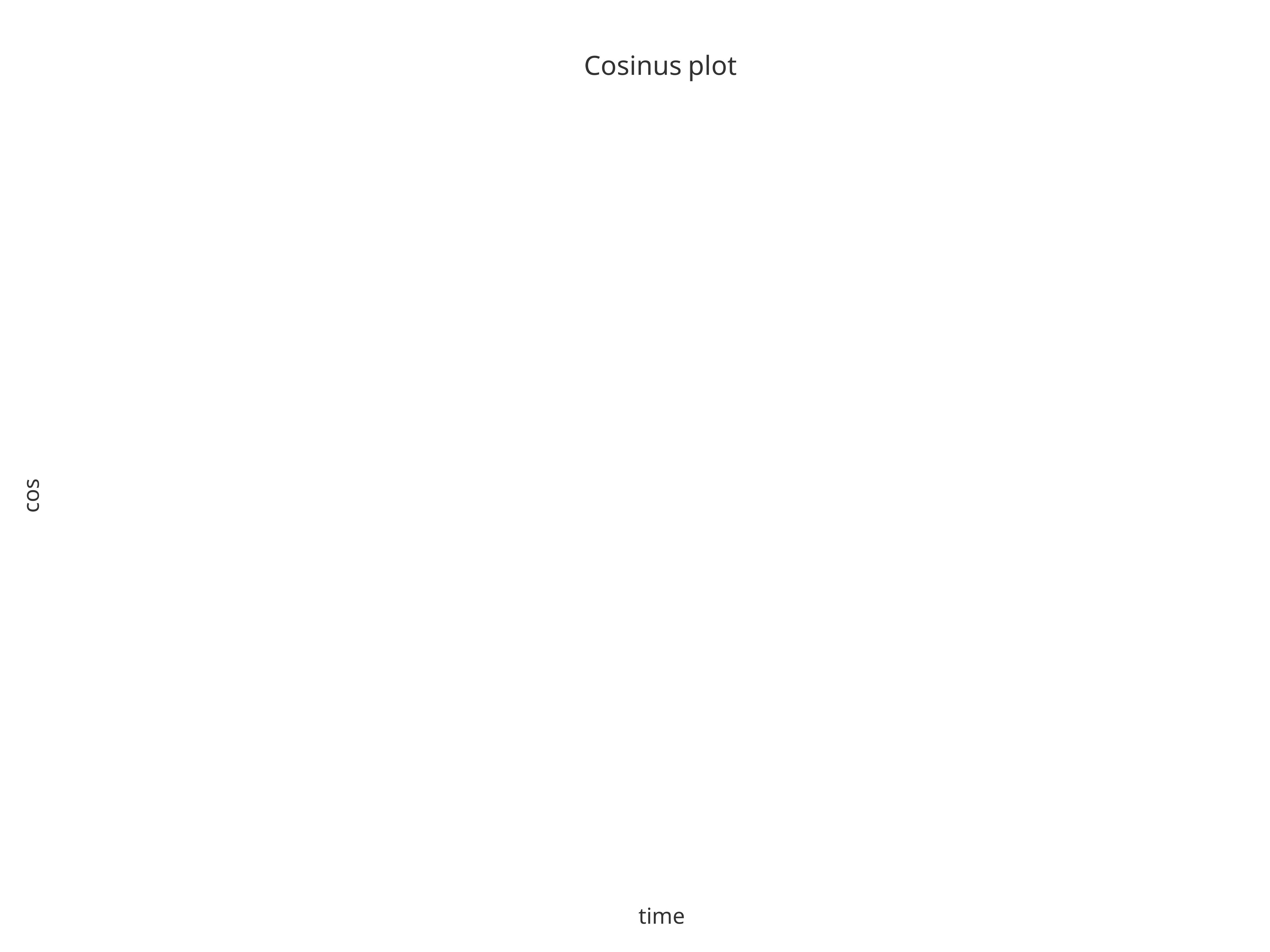# Define default Configuration with metaData
overwriteConfiguration <- PlotConfiguration$new( title = "Cosinus plot", ylabel = "new y label", data = cosData, metaData = cosMetaData, dataMapping = cosMapping ) initializePlot(plotConfiguration = overwriteConfiguration)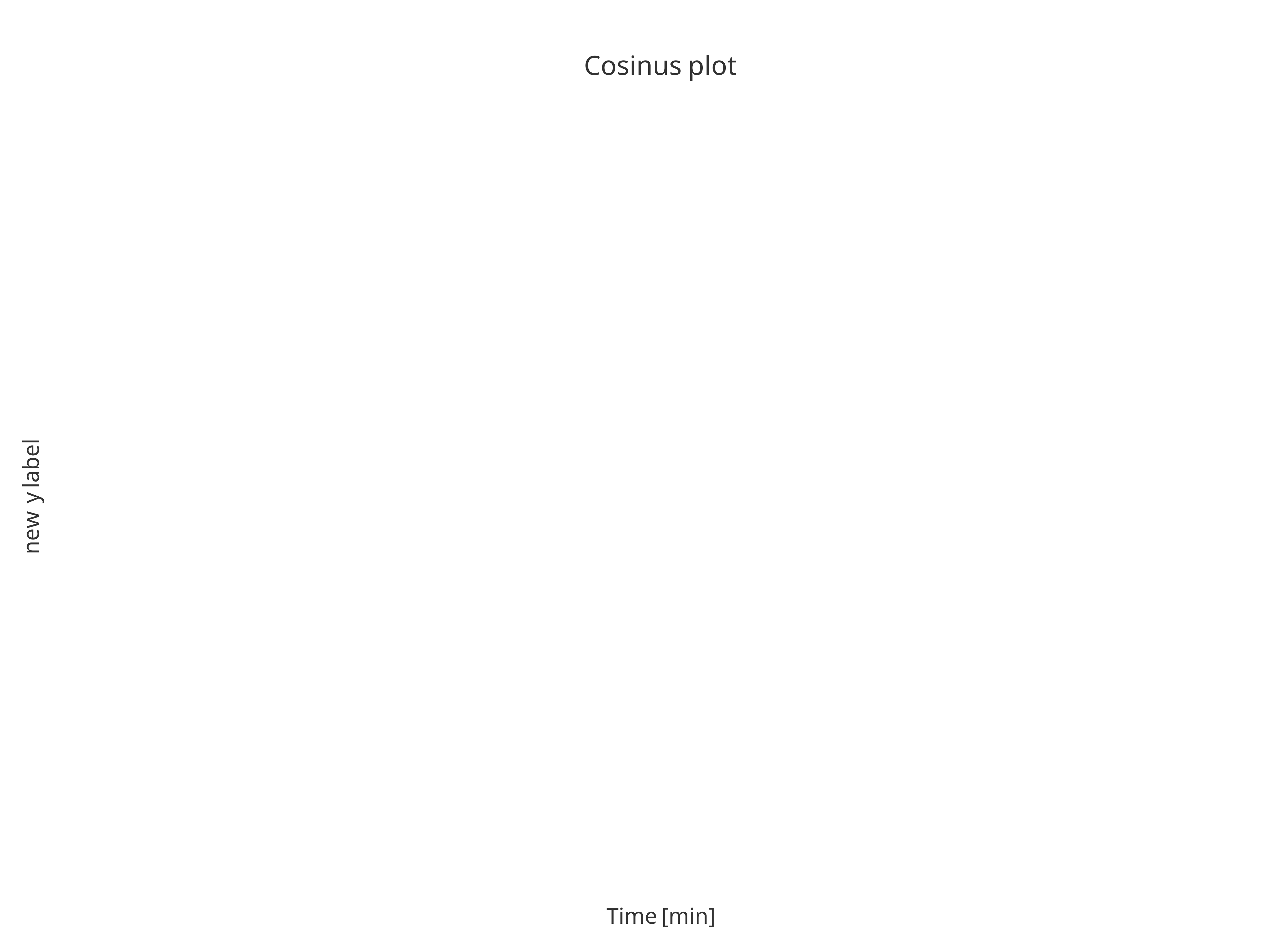## addScatter The function addScatter returns a scatter plot. Likewise all the tlf plot functions, the input argument data and its metaData can be used to defined what to plot. addScatter( data = cosData, metaData = cosMetaData, dataMapping = cosMapping )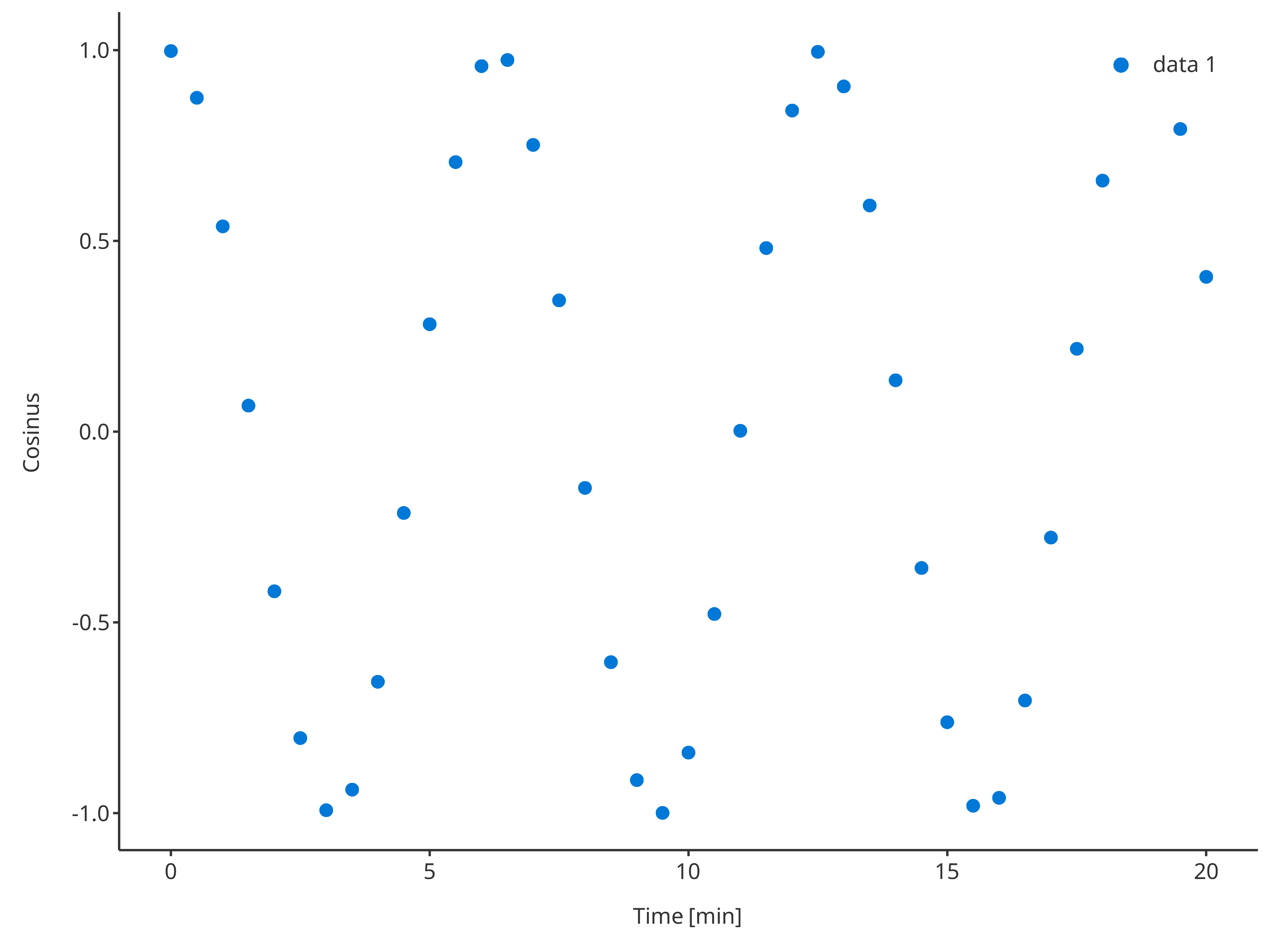The argument plotConfiguration also works the same way as in initializePlot: addScatter( data = cosData, metaData = cosMetaData, dataMapping = cosMapping, plotConfiguration = overwriteConfiguration )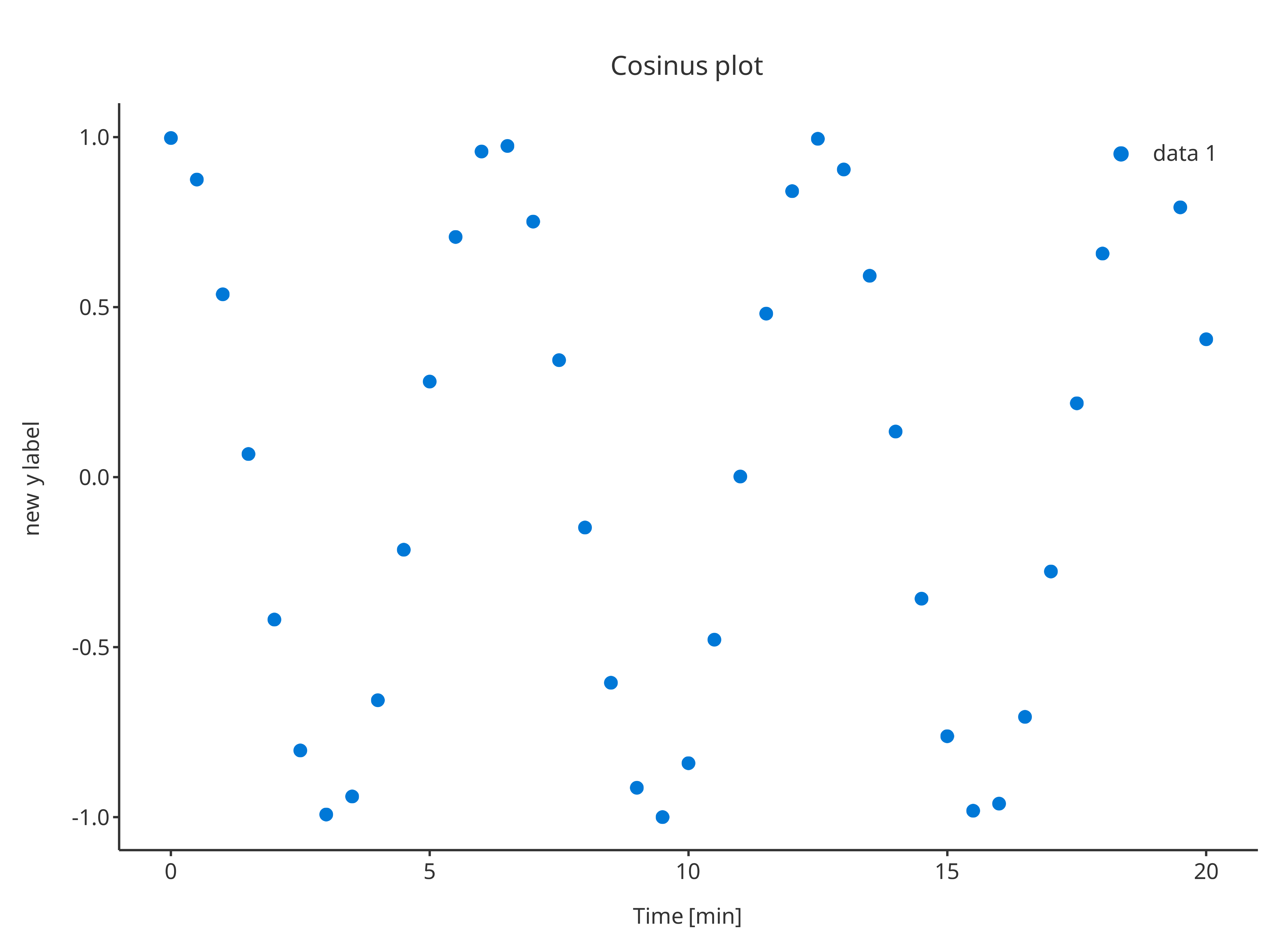The function addScatter also supports x and y inputs instead of data and its dataMapping. In this case, the function will internally create the data.frame with x and y column names along with its data mapping. addScatter( x = cosData$time,
y = cosData$cos )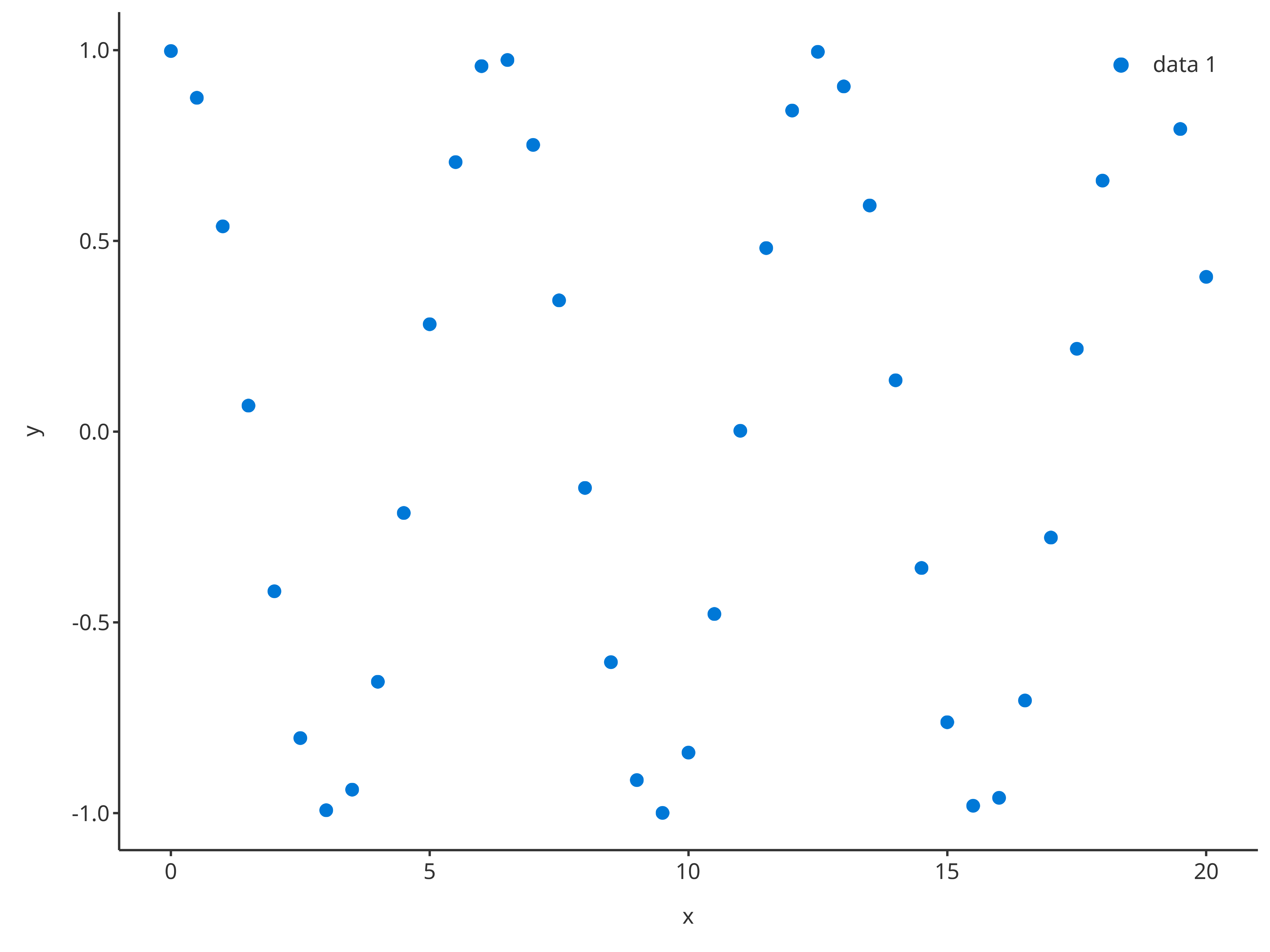It is also possible to prove a plotConfiguration to define the properties especially the labels of the plot created by this process. addScatter( x = cosData$time,
y = cosData$cos, plotConfiguration = overwriteConfiguration )Additionally, optional arguments can be used that will overwrite the default and specific properties of the plotConfiguration. • color: color of the scatter points. Note that multiple colors can be provided if dataMapping defines multiple groups. • shape: shape of the scatter points. Note that multiple shapes can be provided if dataMapping defines multiple groups. • size: size of the scatter points. Note that multiple shapes can be provided if dataMapping defines multiple groups. • linetype: linetype of lines connecting the scatter points. Note that multiple linetypes can be provided if dataMapping defines multiple groups. • caption: Name of the legend caption for the scatter points. Note that multiple captions can be provided if dataMapping defines multiple groups. addScatter( x = cosData$time,
y = cosData$cos, color = "firebrick", shape = Shapes$cardsHeart,
size = 3,
caption = "heart"
)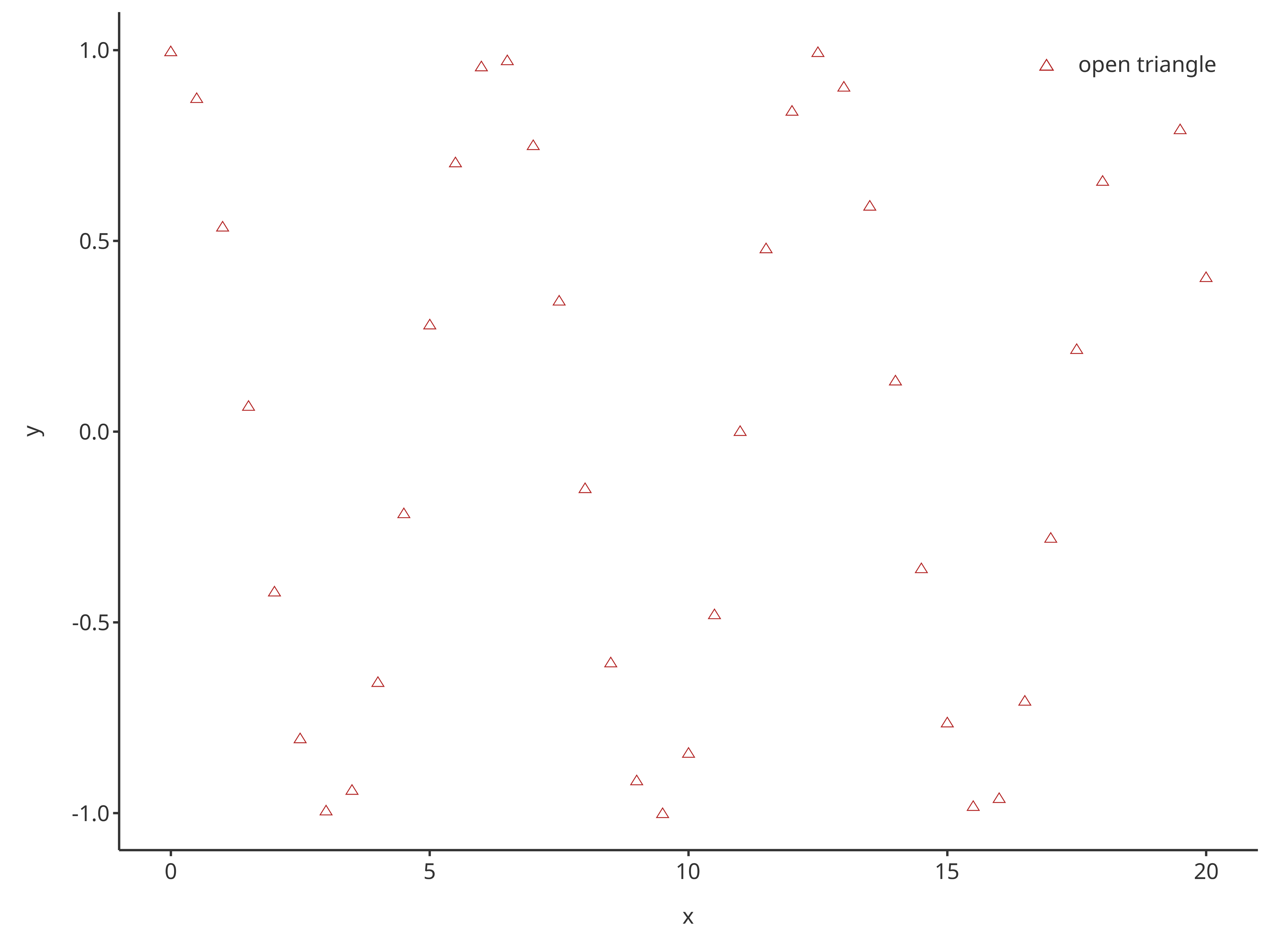If the input argument plotObject is used, then the scatter layer is added on top of the input plot and will use the plotConfiguration of plotObject.

scatterPlot <- addScatter(
x = cosData$time, y = cosData$cos,
color = "firebrick",
shape = Shapes$cardsHeart, size = 3, caption = "heart", plotConfiguration = overwriteConfiguration ) scatterPlot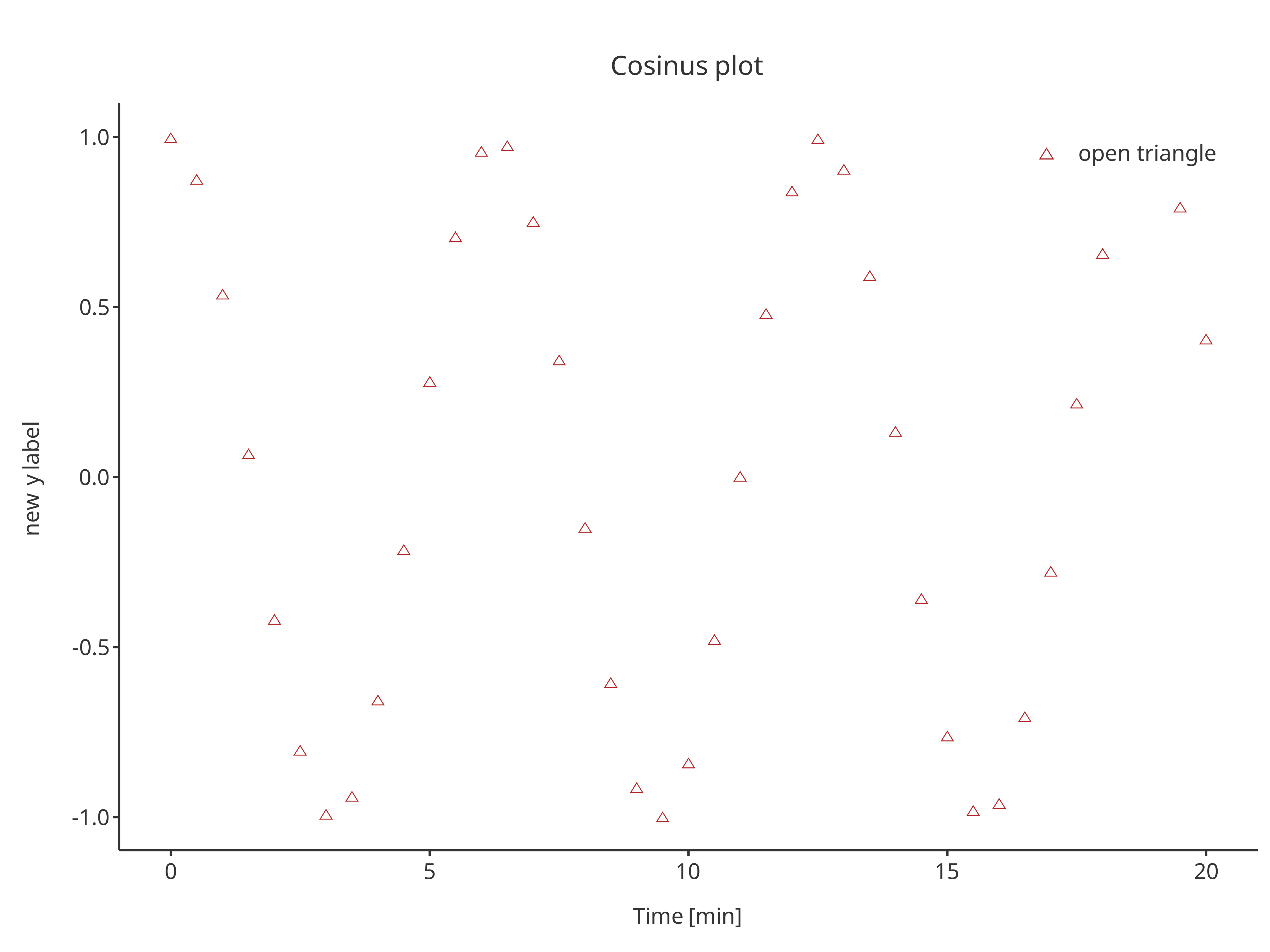addScatter( x = cosData$time,
y = cosData$cos + 2, color = "black", shape = Shapes$cardsSpade,
size = 3,
plotObject = scatterPlot
)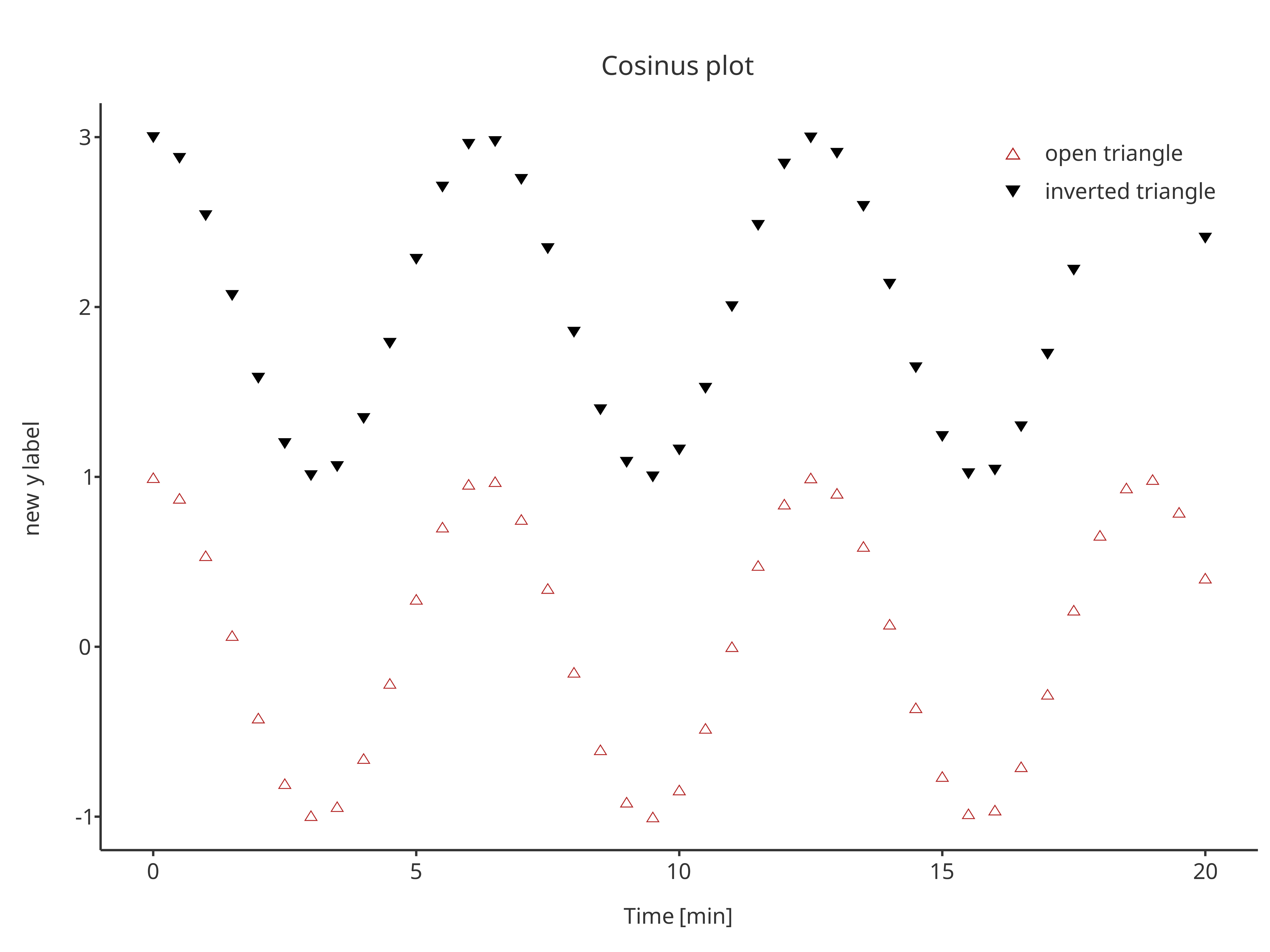## addLine

The function addLine returns a line plot. The same arguments as addScatter can be used by addLine, only default properties are different.

addLine(
x = cosData$time, y = cosData$cos
)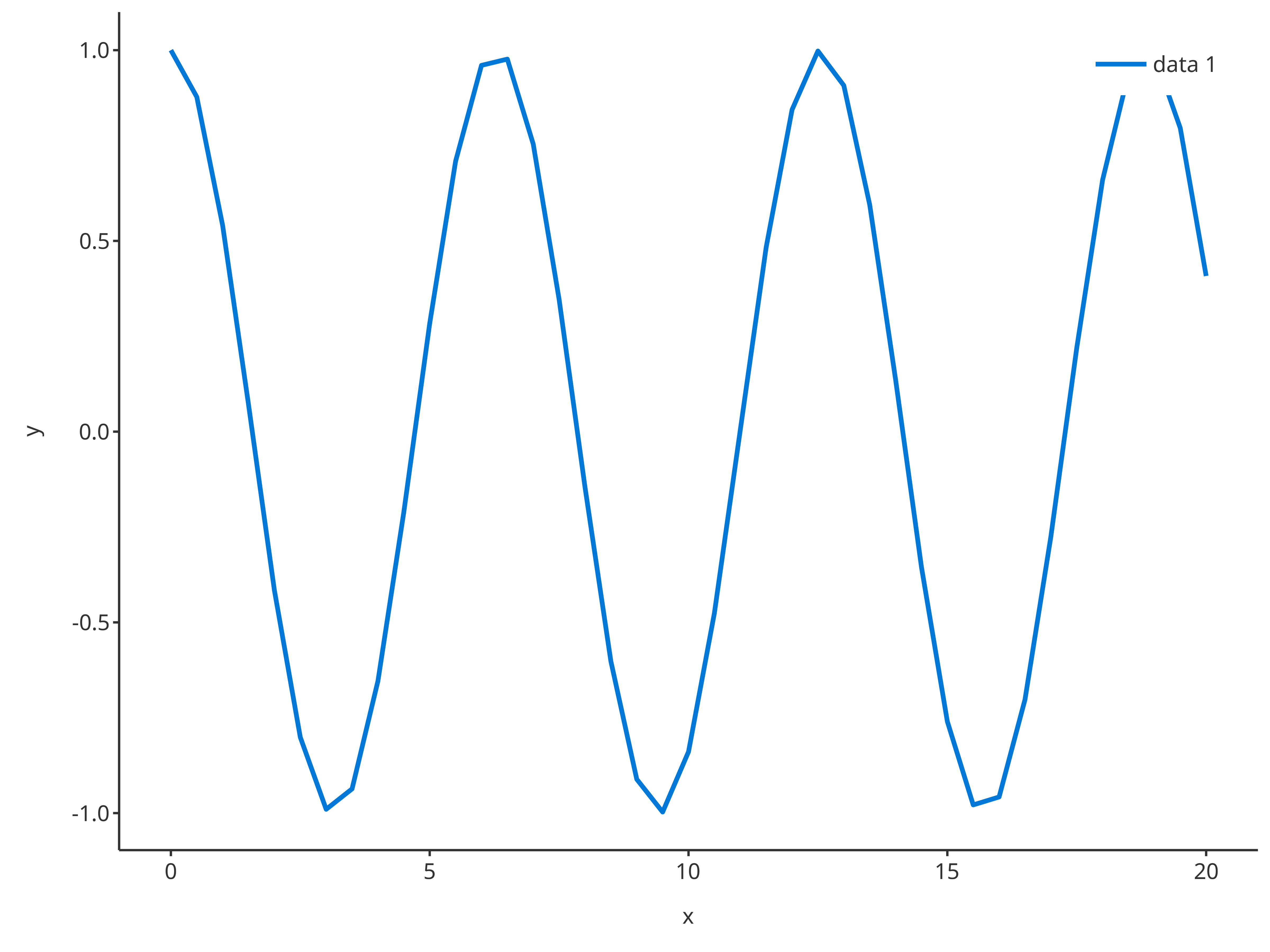addLine(
x = cosData$time, y = cosData$cos,
color = "firebrick",
linetype = Linetypes$longdash, size = 1, caption = "line with\nlong dashes" )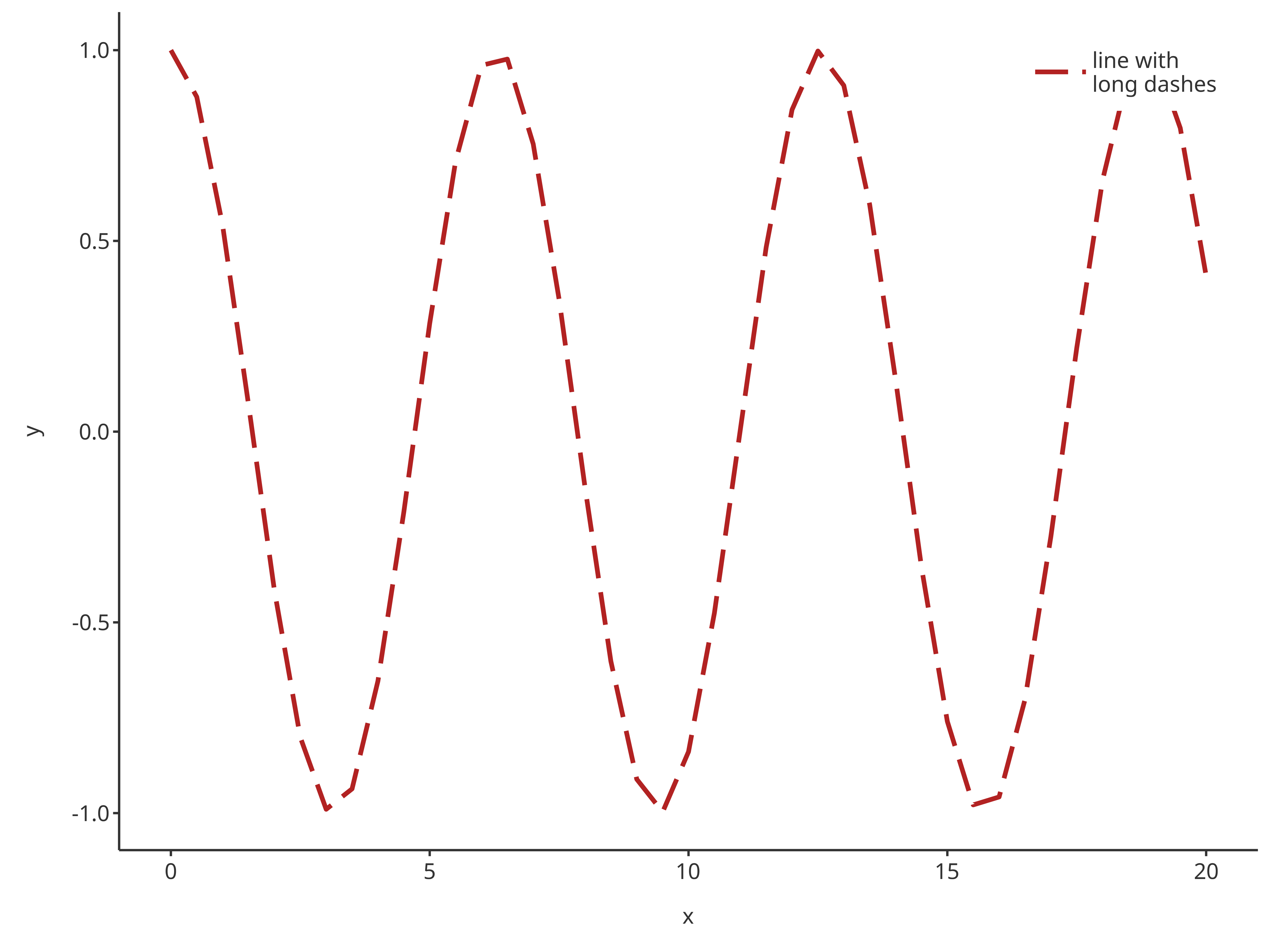Since line plots can inlcude horizontal and vertical lines, addLine has the possibility of using x or y input for x/y-intercepts. addLine( y = c(1, 2, 3), caption = "horizontal lines" ) addLine( x = c(1, 2, 3), caption = "vertical lines" ) The functions addScatter and addLine can also be combined: scatterPlot <- addScatter( x = cosData$time,
y = cosData$cos, color = "firebrick", shape = Shapes$cardsHeart,
size = 3,
caption = "heart",
plotConfiguration = overwriteConfiguration
)

scatterPlot

y = 0.5,
color = "dodgerblue",
linetype = Linetypes$solid, size = 0.5, caption = "threshold", plotObject = scatterPlot ) Caution: It is possible to define the transparency of the points and lines using PlotConfiguration objects using the field alpha in lines or points. Lines with transparency in legend may disappear when the plot is displayed within the viewport for older versions of RStudio (for more details, see https://github.com/tidyverse/ggplot2/issues/4351). However, this does not affect exported plots. ## addRibbon The function addRibbon returns a ribbon plot. Ribbons require ymin and ymax inputs. Consequently, a different dataMapping of type RangeDataMapping is necessary compared to addLine and addScatter that require XYGDataMapping. sinCosMapping <- RangeDataMapping$new(
x = "time",
ymin = "sin",
ymax = "cos"
)

data = cosData,
dataMapping = sinCosMapping
)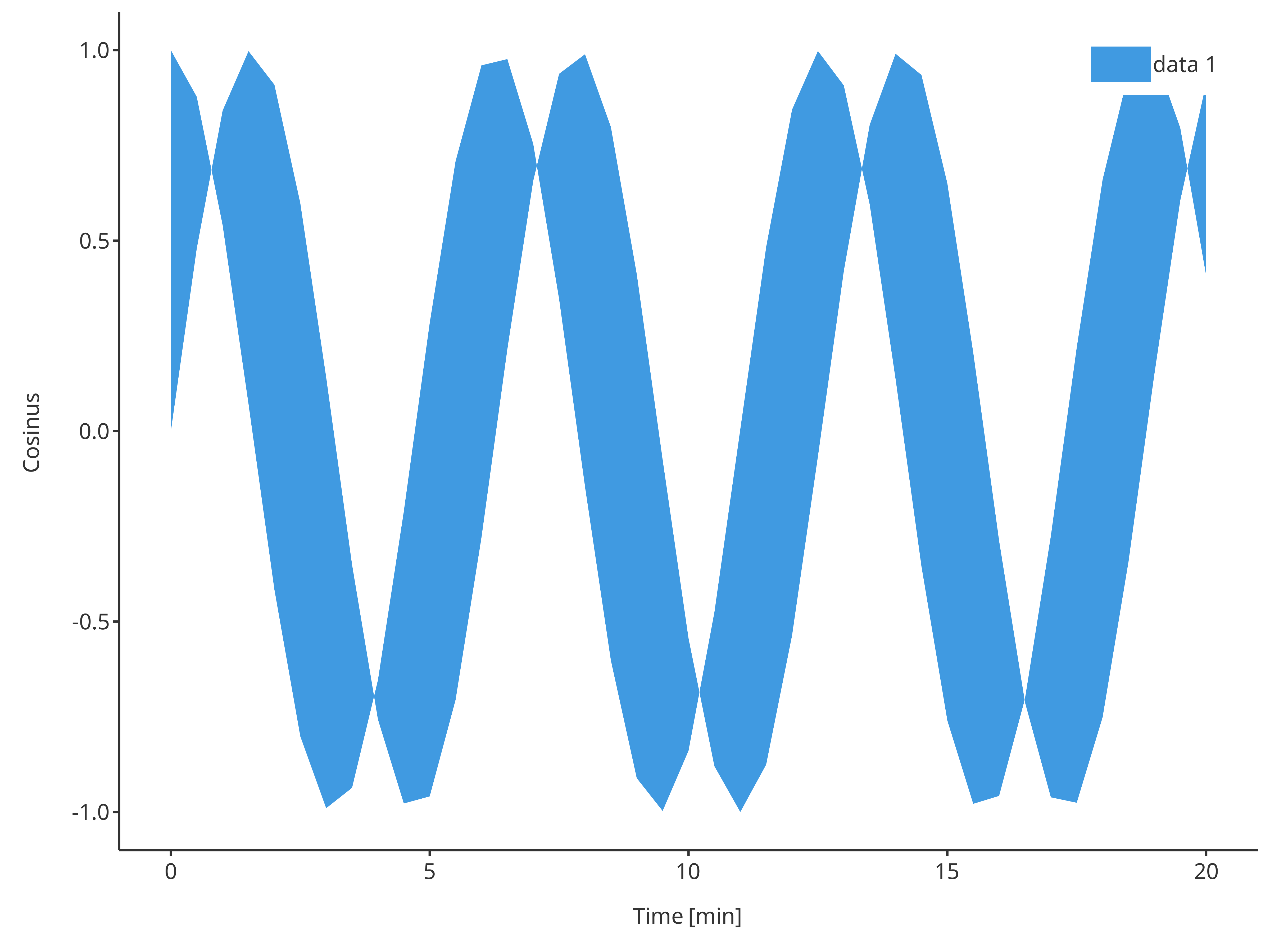The function addRibbon also supports x, ymin and ymax inputs instead of data and its dataMapping.

addRibbon(
x = cosData$time, ymin = cosData$sin,
ymax = cosData$cos )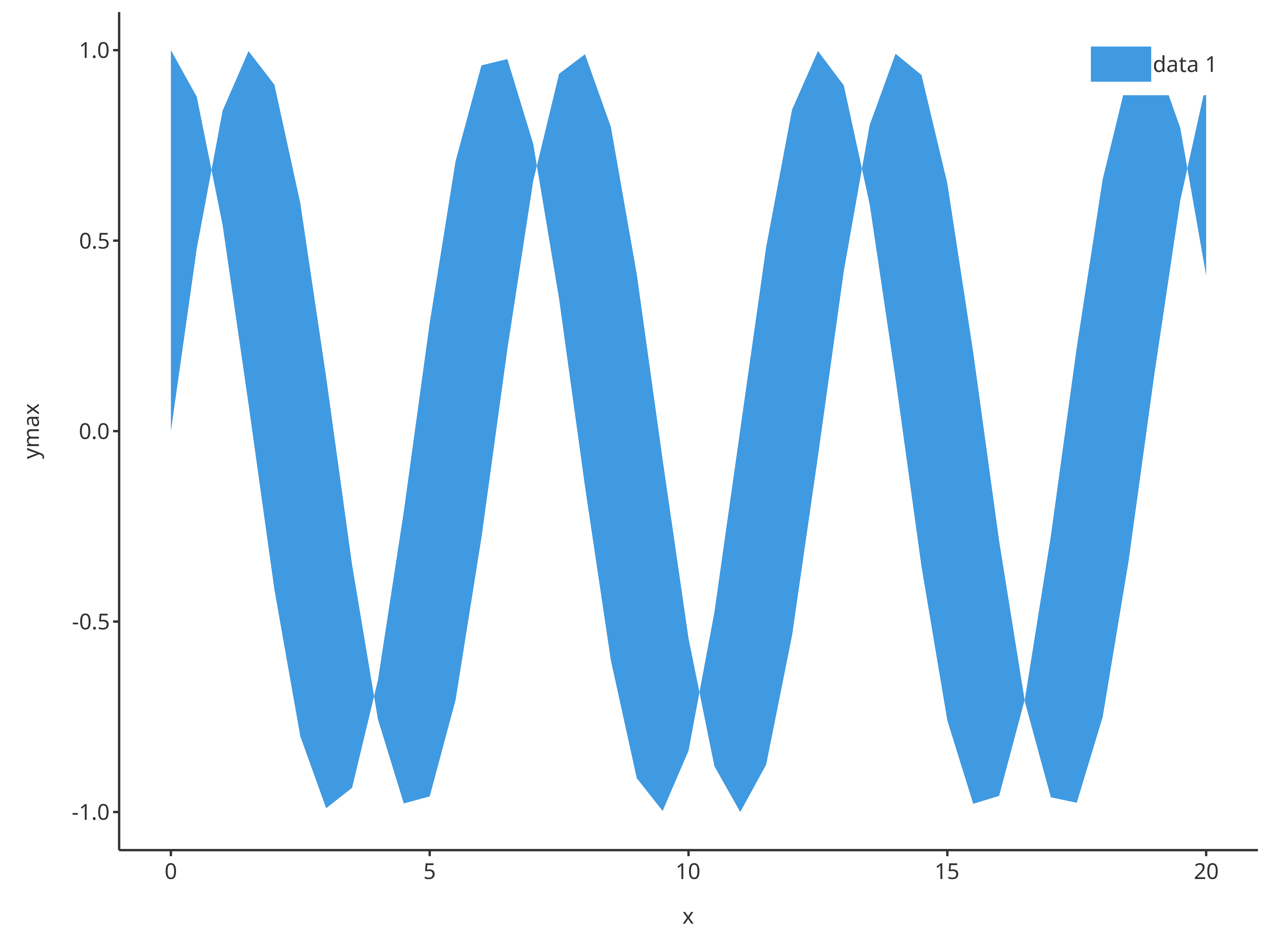For ribbons, optional inputs include fill and alpha defining the color and transparency of the ribbon, respectively. addRibbon( x = cosData$time,
ymin = cosData$sin, ymax = cosData$cos,
fill = "firebrick",
alpha = 0.5,
caption = "ribbon plot"
)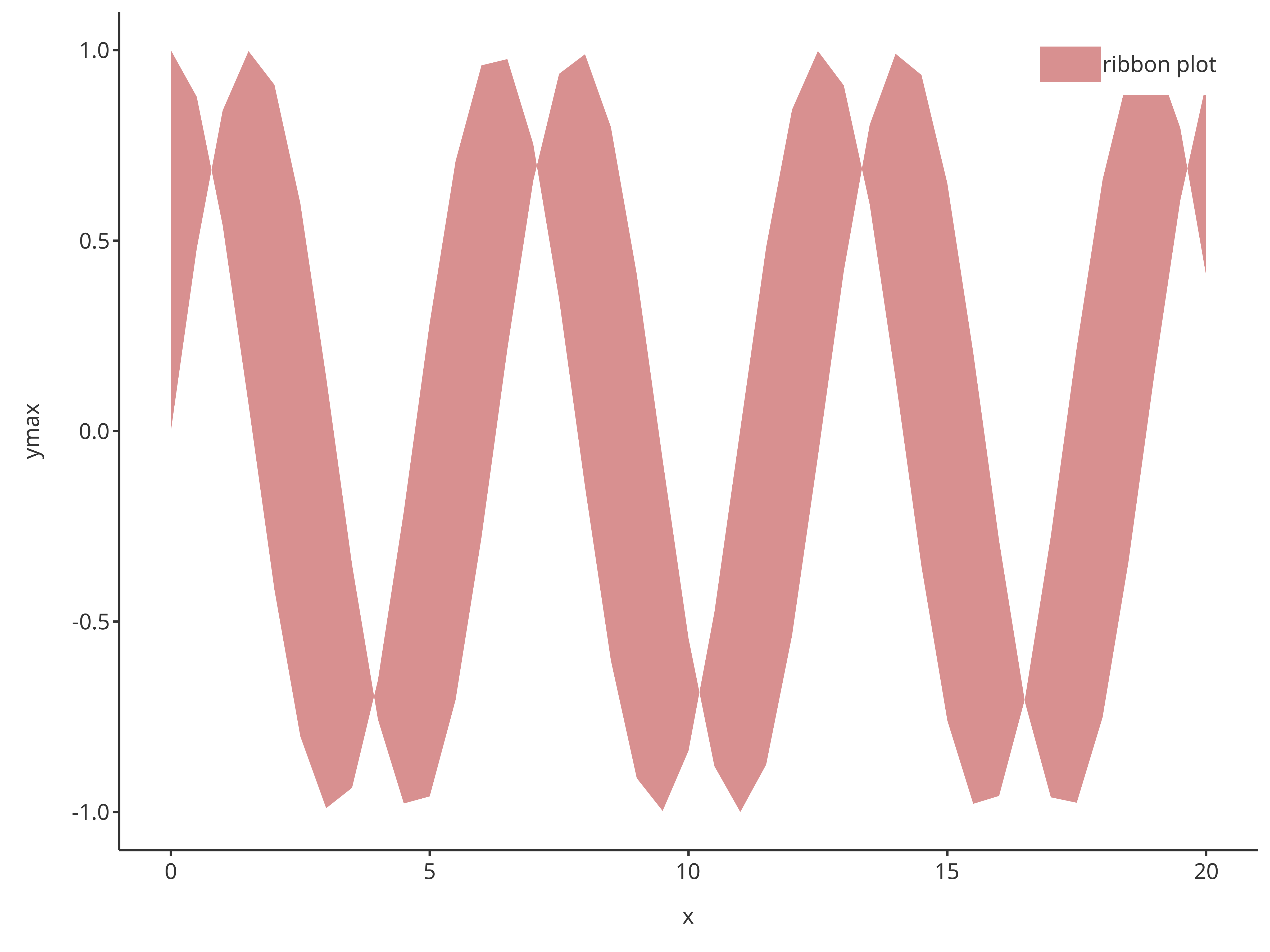Note that transparency input, alpha, is a value between 0 and 1. The closer to 0, the more transparent the fill color is. The closer to 1, the more visible the fill color is.

## addErrorbar

The function addErrorbar returns an error bar plot. As for ribbons, error bars require ymin and ymax inputs.

addErrorbar(
x = cosData$time, ymin = cosData$sin,
ymax = cosData$cos )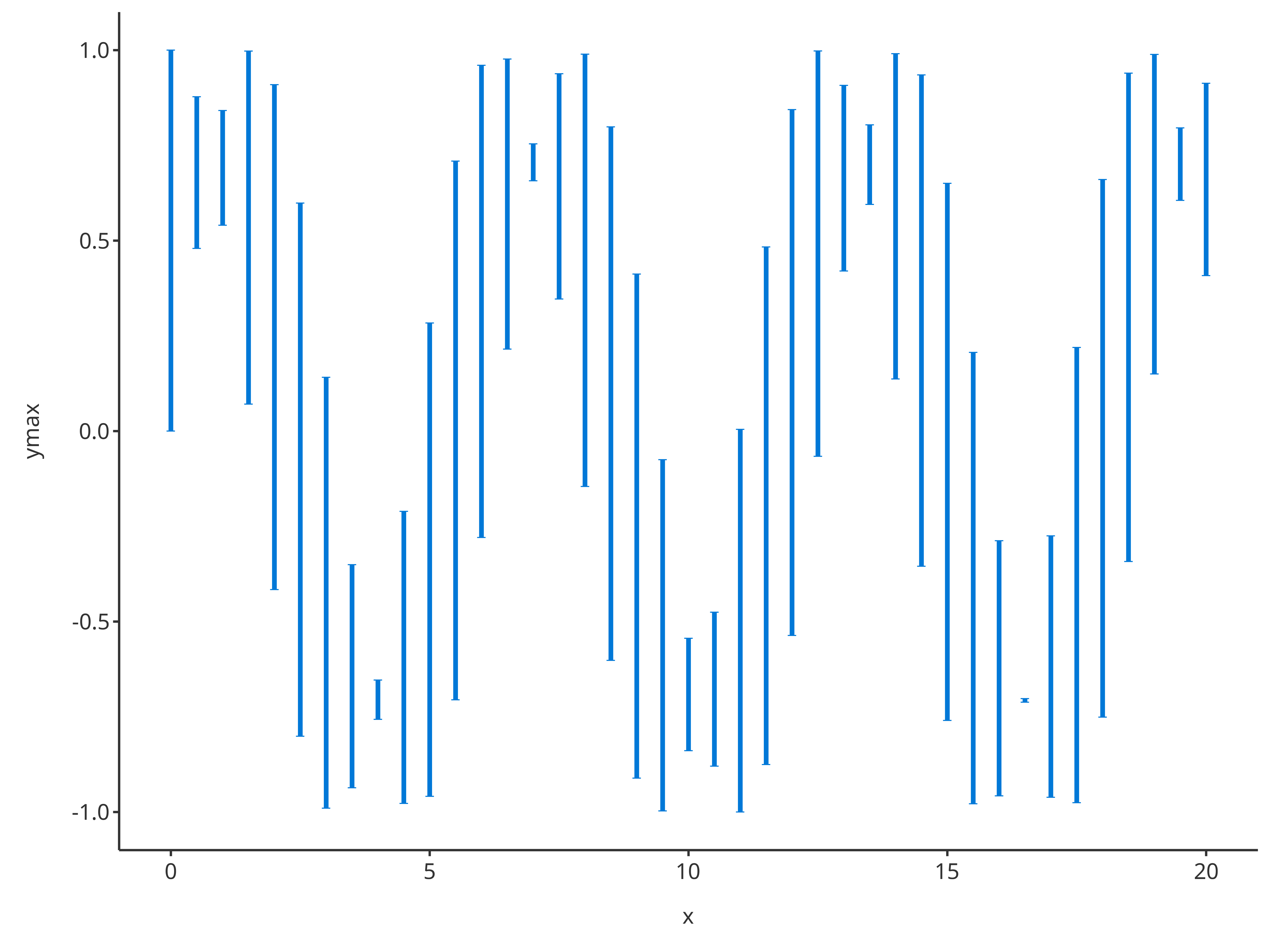Among its optional inputs, addErrorbar proposes includeCap a logical that defines if caps are included at the extremities of the error bars. addErrorbar( x = cosData$time,
ymin = cosData$sin, ymax = cosData$cos,
color = "firebrick",
linetype = Linetypes$solid, includeCap = TRUE, size = 0.5, caption = "error bar plot" )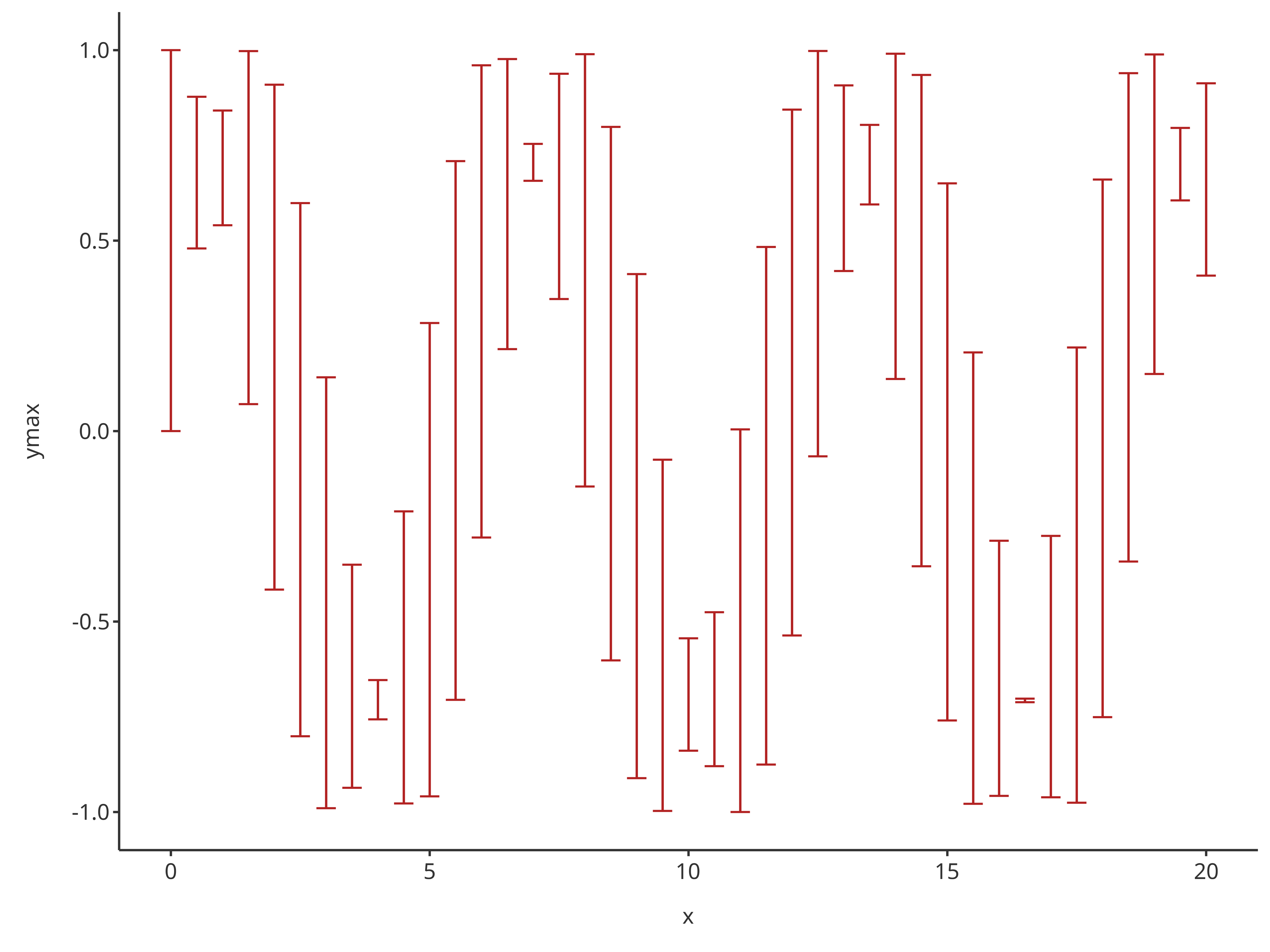Note that optional inputs for addErrorbar define the aesthetic of the bars only (and not how to link the bars). Thus, two layers are necessary when creating a plot that inlcuded both data and their error bars. errorPlot <- addErrorbar( x = cosData$time,
ymin = cosData$cos - 0.1, ymax = cosData$cos + 0.2,
caption = "error",
color = "black",
size = 0.5,
plotConfiguration = overwriteConfiguration
)

errorPlot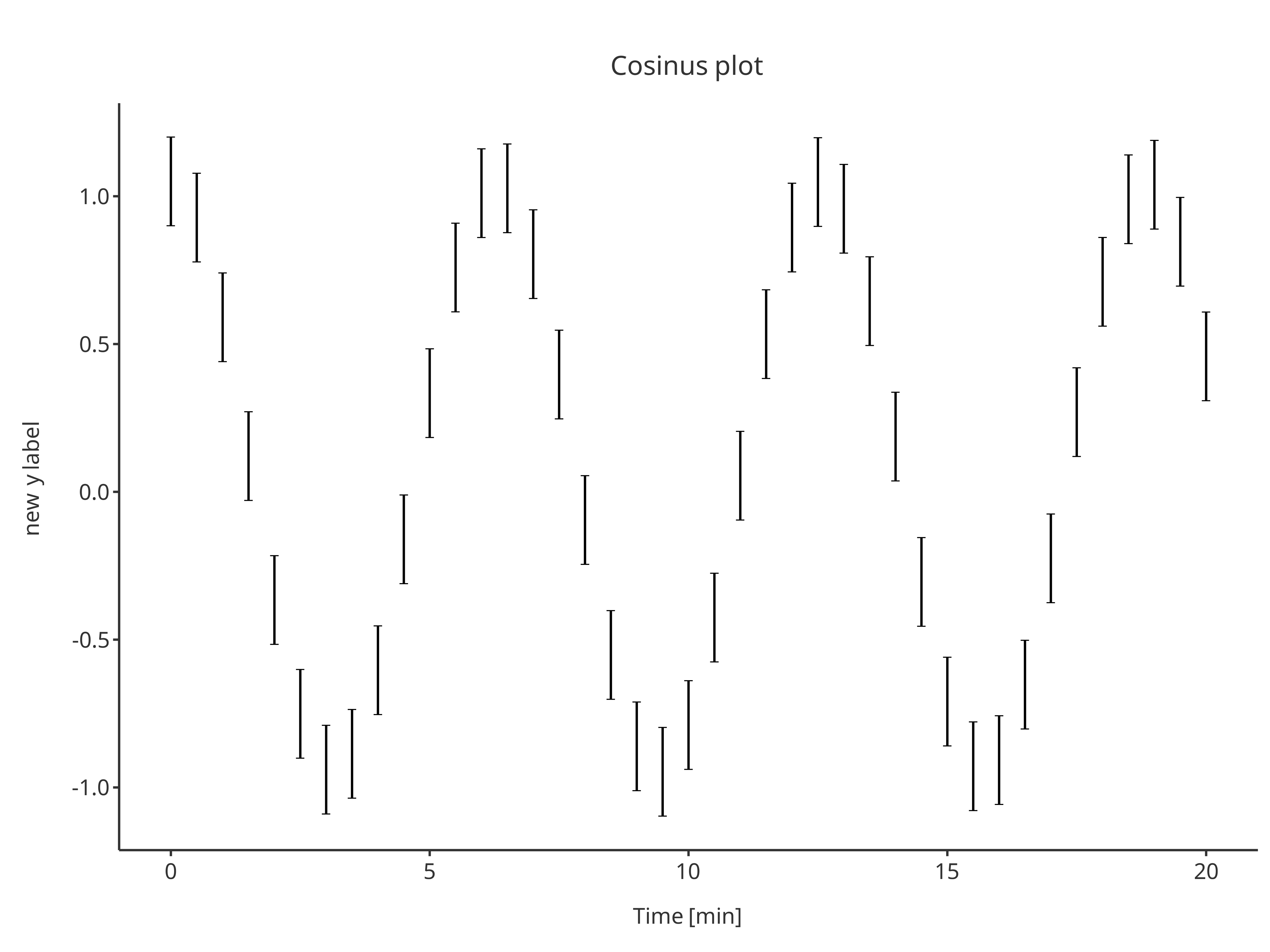x = cosData$time, y = cosData$cos,
)